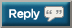# Thread: 2017 Push-up Challenge - try for 40,000 this time

1.2.3.4.5.6.7.8.9.10.11.12. 7,818 + 100 = 791813. + 42 = 796014.15. 8045 + 100 = 814516. 8145 + 170 = 831517. 8315 + 25 = 834018. 8340 + 100 = 844019. 8440+45=848520.21.22.23.24. YTD catch up post:

+ 1,463 = 10,36225. 10,362 + 100 = 10,46226.27.28.29.30.#### Posting Permissions

• You may not post new threads
• You may not post replies
• You may not post attachments
• You may not edit your posts